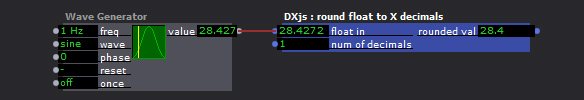# Round to X number of decimals, using Javascript

• I was just answering a support request, where I sent the user a piece of Javascript code I use...

and thought that I should share it here:

It takes in a float and outputs it rounded to the number of decimals set in the second input.

![](http://troikatronix.com/troikatronixforum/discussion/download/2642/jsround.jpg)
```//input 1 = the float val
//input 2 = the number of decimal places to round [0-4]  MUST BE INT

function main()
{
function Nrnd(num, places){
return +(Math.round(num + "e+" + places) + "e-" + places);
}

R = Nrnd(arguments,arguments);
return R;
}
```